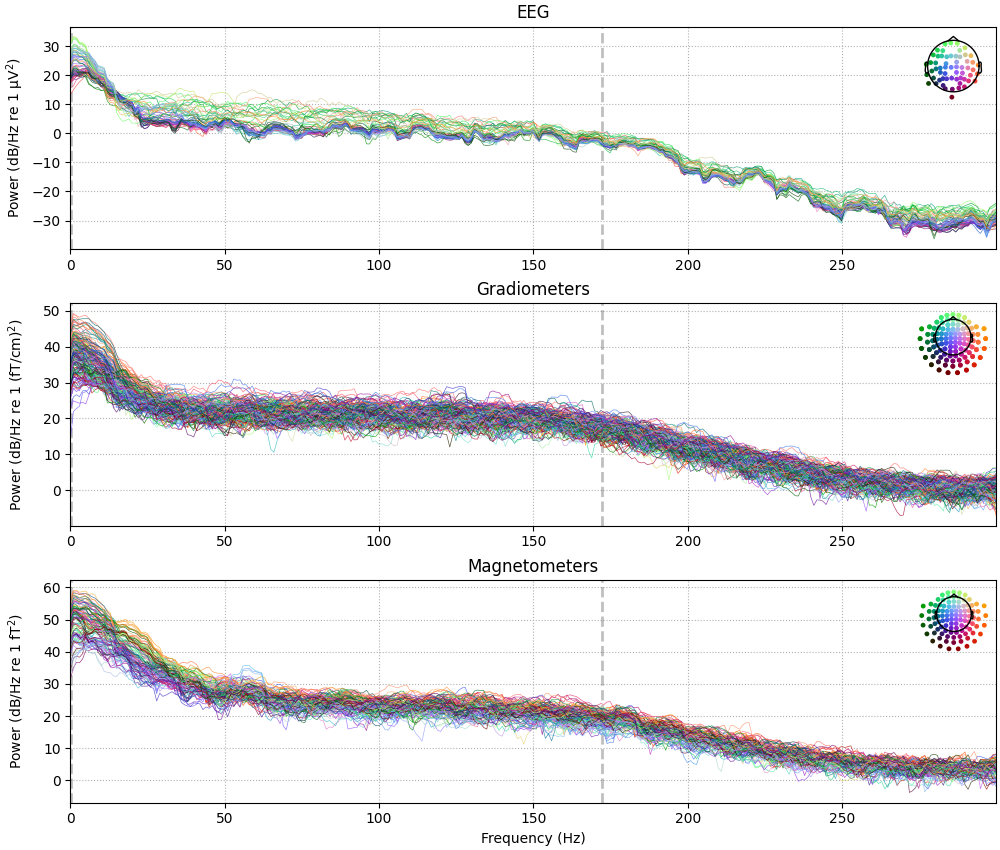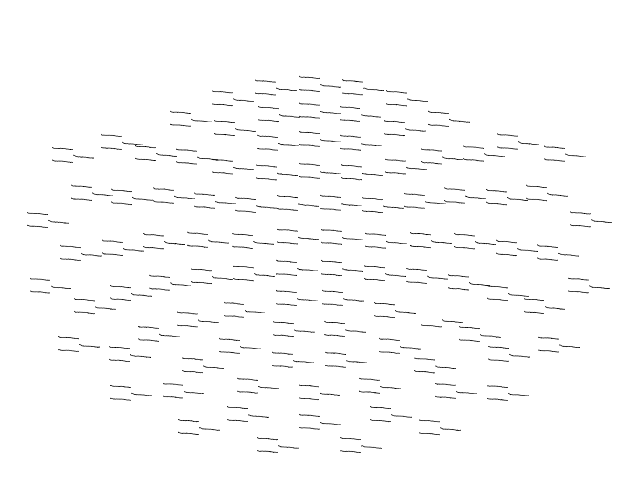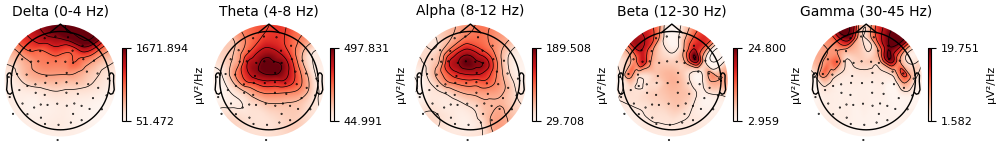# The Spectrum and EpochsSpectrum classes: frequency-domain data#

This tutorial shows how to create and visualize frequency-domain representations of your data, starting from continuous `Raw`, discontinuous `Epochs`, or averaged `Evoked` data.

As usual we’ll start by importing the modules we need, and loading our sample dataset:

```import numpy as np

import mne

sample_data_folder = mne.datasets.sample.data_path()
sample_data_raw_file = sample_data_folder / "MEG" / "sample" / "sample_audvis_raw.fif"
```

All three sensor-space containers (`Raw`, `Epochs`, and `Evoked`) have a `compute_psd()` method with the same options.

```raw.compute_psd()
```
```Effective window size : 3.410 (s)
```
Data type Power Spectrum eeg: V²/Hz grad: (T/m)²/Hz mag: T²/Hz Raw channel, freq welch 364 1025 0.00 – 300.31 Hz

By default, the spectral estimation method will be the Welch method for continuous data, and the multitaper method  for epoched or averaged data. This default can be overridden by passing `method='welch'` or `method='multitaper'` to the `compute_psd()` method.

There are many other options available as well; for example we can compute a spectrum from a given span of times, for a chosen frequency range, and for a subset of the available channels:

```raw.compute_psd(method="multitaper", tmin=10, tmax=20, fmin=5, fmax=30, picks="eeg")
```
```Using multitaper spectrum estimation with 7 DPSS windows
```
Data type Power Spectrum eeg: V²/Hz Raw channel, freq multitaper 59 250 5.10 – 30.00 Hz

You can also pass some parameters to the underlying spectral estimation function, such as the FFT window length and overlap for the Welch method; see the docstrings of `mne.time_frequency.Spectrum` (esp. its `method_kw` parameter) and the spectral estimation functions `psd_array_welch()` and `psd_array_multitaper()` for details.

For epoched data, the class of the spectral estimate will be `mne.time_frequency.EpochsSpectrum` instead of `mne.time_frequency.Spectrum`, but most of the API is the same for the two classes. For example, both have a `get_data()` method with an option to return the bin frequencies:

```with mne.use_log_level("WARNING"):  # hide some irrelevant info messages
events = mne.find_events(raw, stim_channel="STI 014")
event_dict = {
"auditory/left": 1,
"auditory/right": 2,
"visual/left": 3,
"visual/right": 4,
}
epochs = mne.Epochs(
raw, events, tmin=-0.3, tmax=0.7, event_id=event_dict, preload=True
)
epo_spectrum = epochs.compute_psd()
psds, freqs = epo_spectrum.get_data(return_freqs=True)
print(f"\nPSDs shape: {psds.shape}, freqs shape: {freqs.shape}")
epo_spectrum
```
```    Using multitaper spectrum estimation with 7 DPSS windows

PSDs shape: (77, 364, 301), freqs shape: (301,)
```
Data type Power Spectrum eeg: V²/Hz grad: (T/m)²/Hz mag: T²/Hz Epochs 77 epoch, channel, freq multitaper 364 301 0.00 – 299.81 Hz

Additionally, both `Spectrum` and `EpochsSpectrum` have `__getitem__` methods, meaning their data can be accessed by square-bracket indexing. For `Spectrum` objects (computed from `Raw` or `Evoked` data), the indexing works similar to a `Raw` object or a `NumPy array`:

```evoked = epochs["auditory"].average()
evk_spectrum = evoked.compute_psd()
# the first 3 frequency bins for the first 4 channels:
print(evk_spectrum[:4, :3])
```
```NOTE: pick_channels() is a legacy function. New code should use inst.pick(...).
Using multitaper spectrum estimation with 7 DPSS windows
[[5.61863537e-23 1.13487136e-22 9.88010499e-23]
[2.89065956e-23 4.24056397e-23 3.91160668e-23]
[1.56762015e-25 2.65842303e-25 2.39426775e-25]
[5.38758770e-23 1.03771739e-22 9.98519756e-23]]
```

In contrast, the `EpochsSpectrum` has indexing similar to `Epochs` objects: you can use string values to select spectral estimates for specific epochs based on their condition names, and what you get back is a new instance of `EpochsSpectrum` rather than a `NumPy array` of the data values. Selection via hierarchical event descriptors (HEDs) is also possible:

```# get both "visual/left" and "visual/right" epochs:
epo_spectrum["visual"]
```
Data type Power Spectrum eeg: V²/Hz grad: (T/m)²/Hz mag: T²/Hz Epochs 37 epoch, channel, freq multitaper 364 301 0.00 – 299.81 Hz

## Visualizing Spectrum objects#

Both `Spectrum` and `EpochsSpectrum` objects have plotting methods `plot()` (frequency × power), `plot_topo()` (frequency × power separately for each sensor), and `plot_topomap()` (interpolated scalp topography of power, in specific frequency bands). A few plot options are demonstrated below; see the docstrings for full details.

```evk_spectrum.plot(picks="data", exclude="bads")
evk_spectrum.plot_topo(color="k", fig_facecolor="w", axis_facecolor="w")
```
••```evk_spectrum.plot_topomap(ch_type="eeg", agg_fun=np.median)
```## Migrating legacy code#

Below is a quick-reference table of equivalent code from before and after the introduction of the `Spectrum` and `EpochsSpectrum` classes.

Quick reference for common Spectral class actions#

Old

New

`mne.time_frequency.psd_welch(raw)`

`raw.compute_psd().get_data(return_freqs=True)`

`mne.time_frequency.psd_multitaper(raw)`

`raw.compute_psd(method='multitaper').get_data(return_freqs=True)`

`raw.plot_psd(fmin, fmax, dB, area_mode='std')`

`raw.compute_psd(fmin, fmax).plot(dB, ci='std')`

`raw.plot_psd_topo(n_fft, overlap, axes)`

`raw.compute_psd(n_fft, overlap).plot_topo(axes)`

`epochs.plot_psd_topomap(tmax, bands)`

`epochs.compute_psd(tmax).plot_topomap(bands)`

Warning

The functions `mne.time_frequency.psd_welch` and `mne.time_frequency.psd_multitaper` have been removed; new code should use the `Raw.compute_psd()`, `Epochs.compute_psd()`, and `Evoked.compute_psd()` methods, and pass `method='welch'` or `method='multitaper'` as a parameter.

The class methods `Raw.plot_psd()`, `Epochs.plot_psd()`, `Raw.plot_psd_topo()`, and `Epochs.plot_psd_topomap()` have been kept in the API to support legacy code, but should be avoided when writing new code.